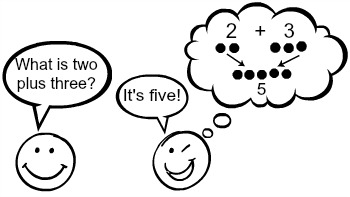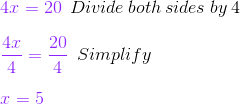# How to Use Mental Math to Solve Equations

Instructor: Laura Pennington

Laura has taught collegiate mathematics and holds a master's degree in pure mathematics.

You may be used to solving arithmetic problems, like addition or subtraction, in your head. In this lesson, we are going to take that mental math a step further and look at how to use mental math to solve equations.

## Mental Math

Suppose I asked you what 2 plus 3 is. Most likely, it wouldn't take you long to tell me that it is 5 without using a calculator or anything else to figure it out. This is called mental math, and we use it to calculate math problems in our heads without having to write anything down or use any tools to do so.

There are many different ways to do mental math. For instance, in the scenario above, I asked you what 2 plus 3 is, and you answered 5. It could be that you have that problem memorized, or it could be that you have a mental trick to figure out what 2 plus 3 is like starting at 2 and then counting three more, or picturing 2 objects and 3 more objects, then counting them all up.Whichever way you choose to do mental math, you are probably familiar with being able to add, subtract, multiply, or divide in your head without too much difficulty, especially with smaller numbers.

Now suppose I ask you what number, when multiplied by 4, gives you 20? Hmmm, that's a little trickier. Basically, I'm asking you for a number, x, that when multiplied by 4 gives 20, or what is x if 4x = 20? Ah-ha! You may recognize that as an equation, making the problem a bit more clear.

## Equations

Equations are statements in mathematics that are made by putting two mathematical expressions equal to each other. Consider our equation 4x = 20. We can use algebra to solve this equation by dividing both sides of the equation by 4.We see that x = 5. So, the number, that when multiplied by 4, gives 20, is 5. We call x = 5 the solution to the equation. In general, a solution to an equation is a number that when plugged in for the variable, in our case x, makes the equation a true statement.

## Solving Simple Equations Using Mental Math

You may be familiar with solving equations using algebra as we just did, but I've got some exciting news! We can actually solve simple equations using mental math. Remember how we set up our equation from the statement 'a number that when multiplied by 4 gives 20'. These types of statements are the key to solving equations mentally. To solve an equation using mental math, we use the following steps:

1. Convert the equation into words.
2. Put those words in the form of a question, and answer the question using inverse operations.

So, what do you think this means? Well, consider our earlier example again. The equation 4x = 20 can be put into words by saying 'a number that when multiplied by 4 gives 20.' We put that into question form by asking 'what number times 4 equals 20?' That question is probably pretty easy for you to answer! It's 5!

If the answer to the question isn't obvious to you, you can make use of inverse operations to reword the question. Inverse operations are basically operations that are opposites of each other. In other words, the inverse operation of addition is subtraction and vice versa, and the inverse operation of multiplication is division and vice versa.

Let's consider some simple examples of equations involving addition, subtraction, multiplication, and division and see the question we want to ask when solving these types of equations mentally.

Operation Addition Subtraction Multiplication Division
Equation x + 2 = 9 x - 7 = 3 3x = 15 x / 2 = 11
Words A number plus 2 equals 9 A number minus 7 equals 3 A number times 3 equals 15 A number divided by 2 equals 11
Question What number plus 2 equals 9? What number minus 7 equals 3? What number times 3 equals 15? What number divided by 2 equals 11?
Inverse Question What is 2 subtracted from 9? (9-2) What is 7 added to 3? (3+7) What is 3 divided into 15? (15/3) What is 2 multiplied by 11? (11*2)
Answer 9 - 2 = 7 3 + 7 = 10 15 / 3 = 5 11*2 = 22

We see that we can put an equation into question form and answer it, or if the answer isn't immediately obvious, we can use inverse operations to reword the question and answer it that way.

To unlock this lesson you must be a Study.com Member.

### Register to view this lesson

Are you a student or a teacher?

### Unlock Your Education

#### See for yourself why 30 million people use Study.com

##### Become a Study.com member and start learning now.
Back
What teachers are saying about Study.com

### Earning College Credit

Did you know… We have over 160 college courses that prepare you to earn credit by exam that is accepted by over 1,500 colleges and universities. You can test out of the first two years of college and save thousands off your degree. Anyone can earn credit-by-exam regardless of age or education level.